Function Repository Resource:

# EulerianNumber

Get the number of permutations with a given number of ascents

Contributed by: Wolfram Staff
 ResourceFunction["EulerianNumber"][n,k] gives the number of permutations of {1,2,…,n} with k ascents.

## Details and Options

The index i of a permutation written as p1,p2,,pn in one-line notation is an ascent if pi<pi+1.

## Examples

### Basic Examples (3)

Count the number of ascents of the 24 permutations of {1,2,3,4}:

 In:=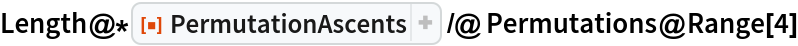Out=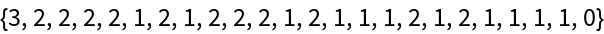Tally up permutations by the number of ascents:

 In:=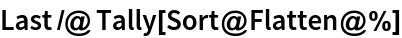Out=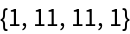EulerianNumber gives the same list, calculated without implicitly listing the individual ascents:

 In:=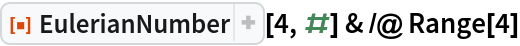Out=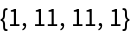### Scope (2)

The numbers form an infinite lower-triangular matrix:

 In:=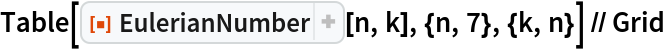Out=The row sums are the factorials because they count the number of permutations:

 In:=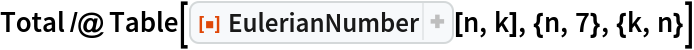Out=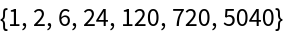In:=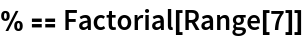Out=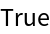### Neat Examples (4)

Here the entries are signed in a checkerboard pattern:

 In:=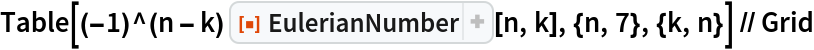Out=Here are those row sums:

 In:=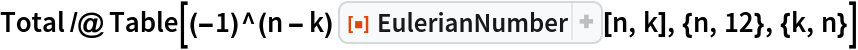Out=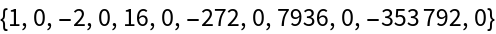Expand Tan[x]:

 In:=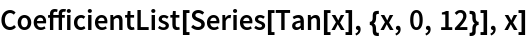Out=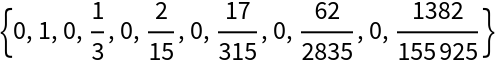Get rid of the factorials in the denominators to match the row sums up to signs and a shift:

 In:=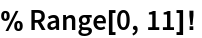Out=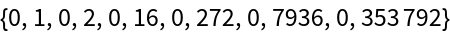George Beck

## Version History

• 3.0.0 – 28 June 2019
• 2.0.0 – 28 March 2019
• 1.0.0 – 15 March 2019